### Lighter or Heavier – Measurement Worksheets Grade 1

Q.1) The  lighter animal amongst the following animal is  ?​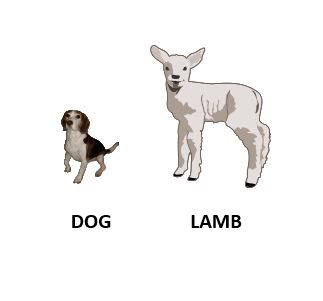[A] Lamb​

[B] Dog​

Q.2) ​The  heavier animal amongst the following animal is  ?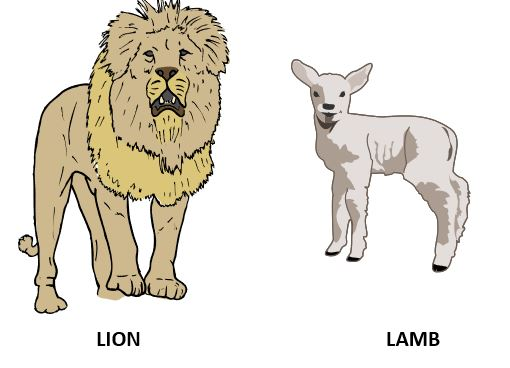[A] ​Lion

[B] ​Lamb

Q.3) The  lighter vegetable amongst the following vegetable is  ?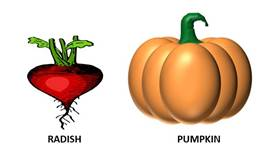[A]  Pumpkin

Q.4) The  lighter vegetables amongst the following vegetables is ?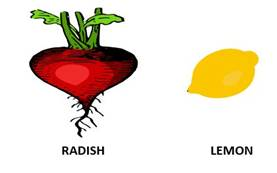[B] Lemon

Q.5) The  heavier vegetable amongst the following vegetable is  ?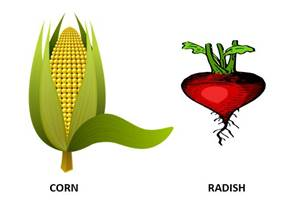[A] C​orn

Q.6) The  lightest vegetable amongst the following vegetable is  ?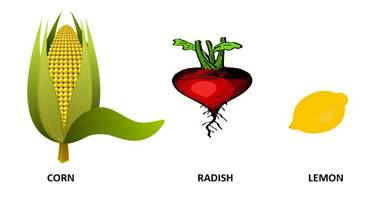[A] Corn

[C] Lemon

Q.7) The  heavier vegetable amongst the following vegetable is  ?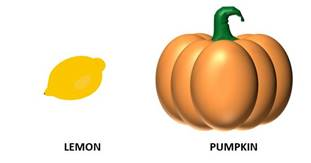[A] Lemon

[B] Pumpkin​

Q.8) ​The  heavier animal amongst the following animal is  ?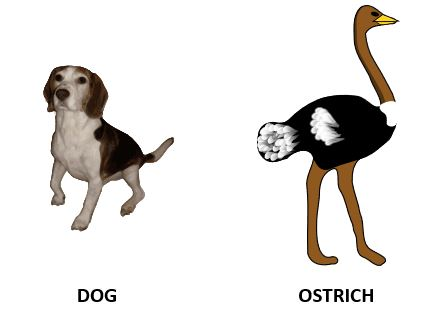[A] Dog​

[B] ​Ostrich

Q.9) ​The  heaviest animal amongst the following animal is  ?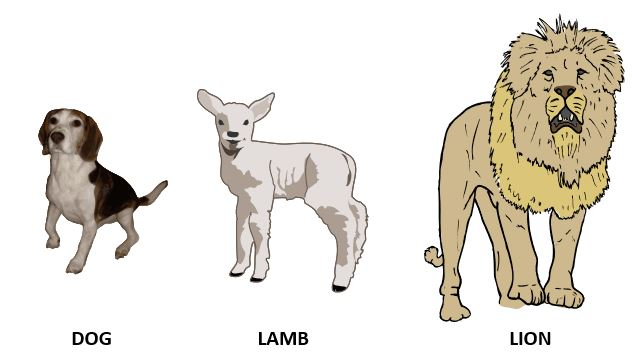[A] ​Dog

[B] ​Lamb

[C] ​Lion

Q.10) ​The  lighter animal amongst the following animal is  ?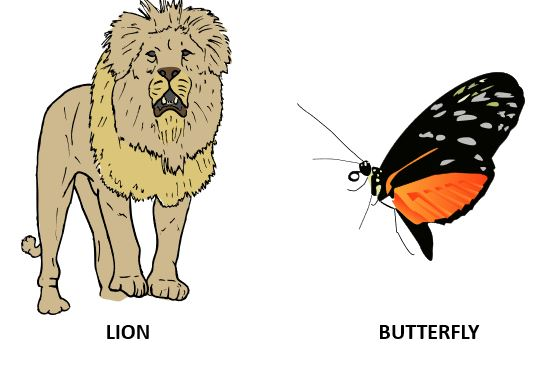[A] Lion​

[B] Butterfly​

### Q.1) ​The lighter object amongst the following object is  ?[A] ​1

[B] ​2

Q.2) ​The  lighter object amongst the following object is  ?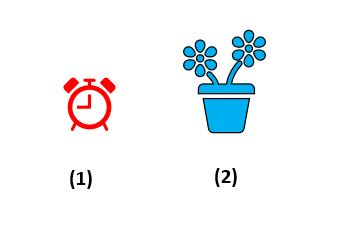[A] 1​

[B] 2​

Q.3) ​The  heavier object amongst the following object is  ?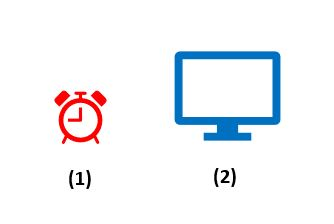[A] ​1

[B] ​2

Q.4) ​The  lighter object amongst the following object is  ?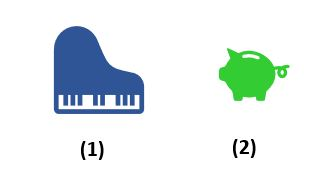[A] 1​

[B] 2​

Q.5) ​The  heavier object amongst the following object is  ?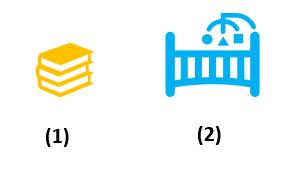[A] ​1

[B] ​2

Q.6) ​The  lighter object amongst the following object is  ?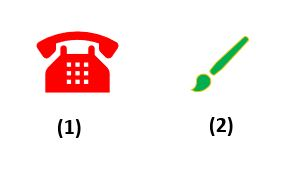[A] 1​

[B] 2​

Q.7) The  lighter object amongst the following object is  ?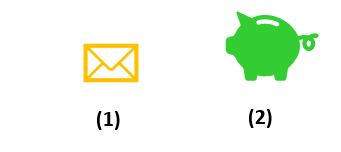[A] 1​

[B] 2​

Q.8) The  heavier object amongst the following object is  ?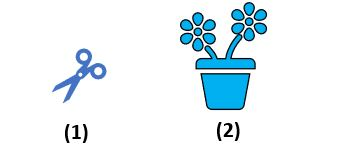[A] 1​

[B] 2​

Q.9) ​The  lighter object amongst the following object is  ?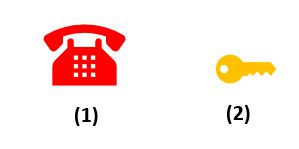[A] ​1

[B] ​2

Q.10) ​The  lighter object amongst the following object is  ?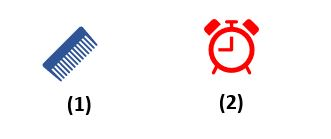[A] 1​

[B] 2​

### Longer or Shorter – Measurement Worksheets Grade 1

Q.1) The longest finger in the hand is ?​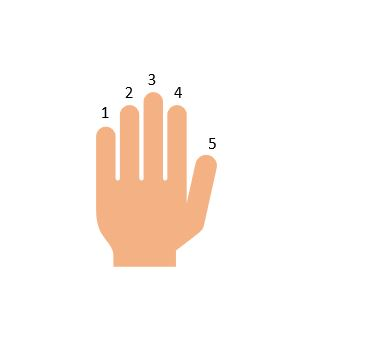[A]  ​1

[B] ​ 2

[C]  3

[D]  4

[E]  ​5

Q.2) The shorter finger in the hand is ?​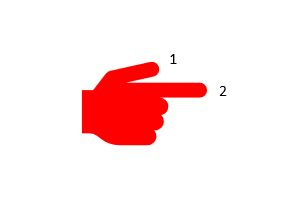[A] ​1

[B] ​2

Q.3) ​Which is  shorter   ? ​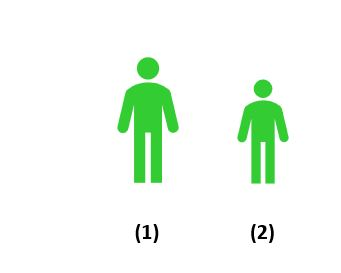[A] ​1

[B] ​2

Q.4) ​Which is  longer ?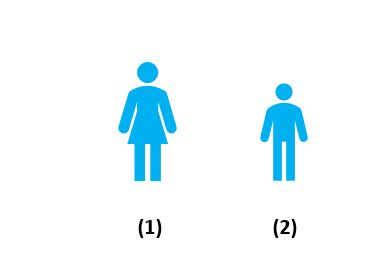[A] ​1

[B] ​2

Q.5) ​Which is  the longest ?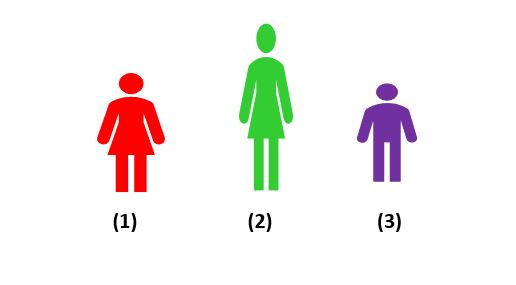[A]  ​1

[B] ​2

[C]  ​3

Q.6) ​Which is  longer? ​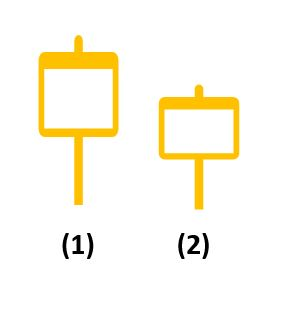[A] ​1

[B] ​2

Q.7) ​Which is  shorter ?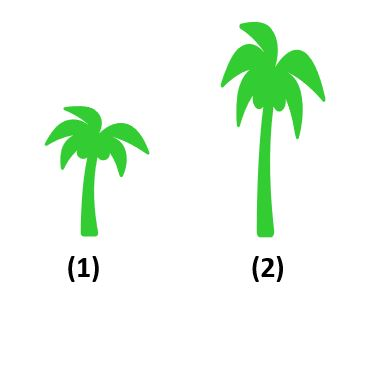[A] ​1

[B] ​2

Q.8) ​Which is  the shortest  ?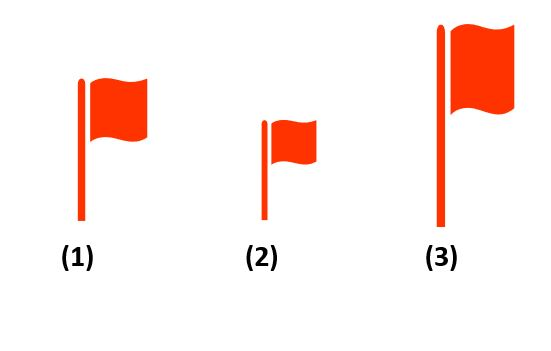[A] ​1

[B] ​2

[C] ​3

Q.9) ​Which is  longer ?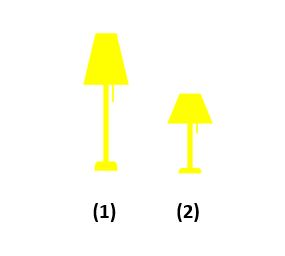[A] ​1

[B] ​2

Q.10) ​Which is  shorter ?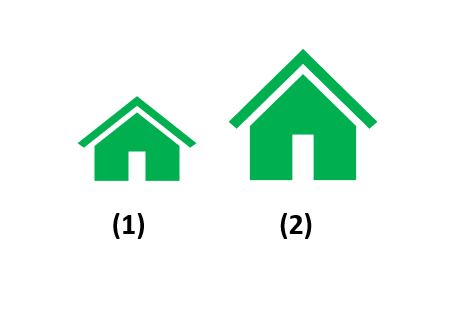[A] ​1

[B] 2​

### Greater and Smaller Capacity – Measurement Worksheets Grade 1

Q.1) ​Which of the following has a greater capacity?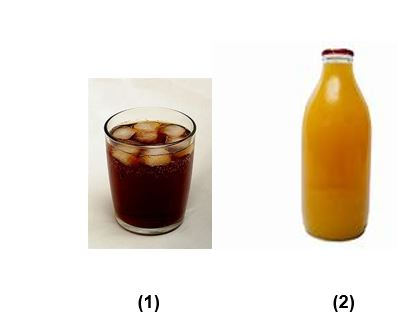[A] ​1

[B] ​2

Q.2) ​Which of the following has a smaller capacity?​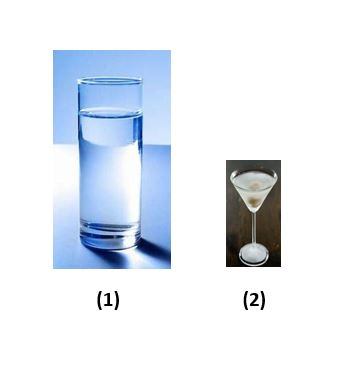[A] ​1

[B] ​2

Q.3) ​Which of the following has a greater capacity?​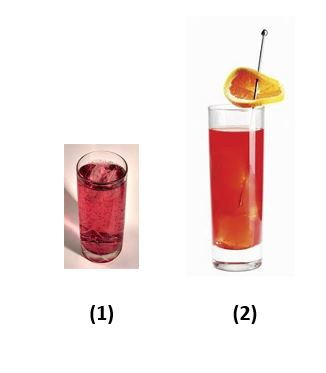[A] ​1

[B] ​2

Q.4) ​Which of the following has a greater capacity?​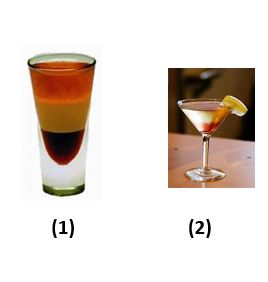[A] ​1

[B] ​2

Q.5) Which of the following has a smaller capacity?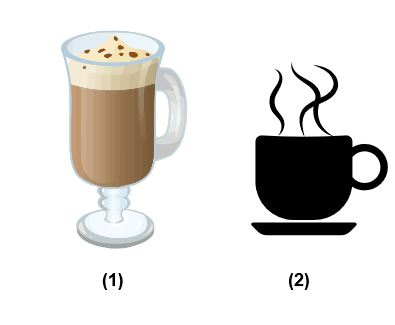​[A] ​1

[B] ​2

Q.6) ​Which of the following has a greater capacity?​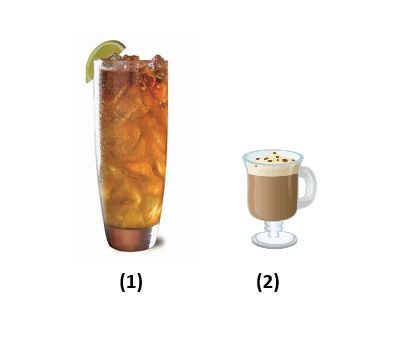[A] ​1

[B] ​2

Q.7) ​Which of the following has a smaller capacity?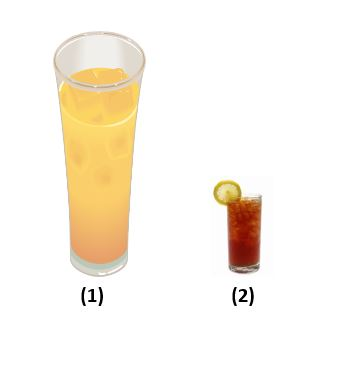[A] ​1

[B] ​2

Q.8) ​Which of the following has the greatest capacity?​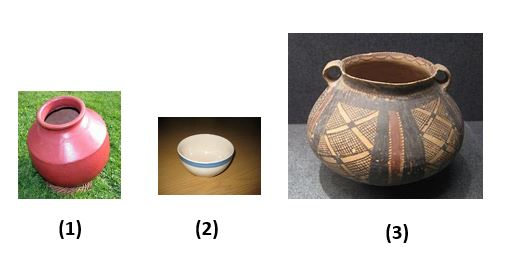[A] ​1

[B] ​2

[C] ​3

Q.9) ​Which of the following has the greatest capacity?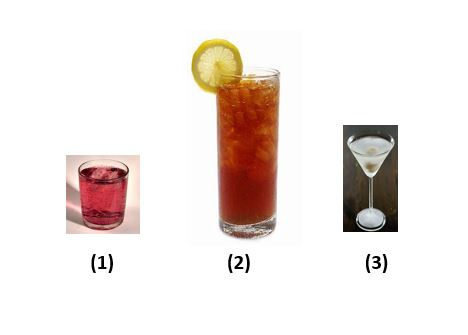[A] ​1

[B] ​2

[C] ​3

Q.10) ​Which of the following has a smaller capacity?​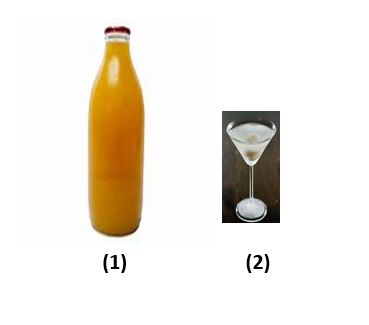[A] ​1

[B] ​2

Q.1)B
Q.2)A
Q.3)B
Q.4)B
Q.5)A
Q.6)C
Q.7)B
Q.8)B
Q.9)C
Q.10)B

Answers – Lighter or Heavier ( Objects ) – Measurement Worksheets Grade 1

Q.1)A
Q.2)A
Q.3)B
Q.4)B
Q.5)B
Q.6)B
Q.7)A
Q.8)B
Q.9)B
Q.10)A

Q.1)C
Q.2)A
Q.3)B
Q.4)A
Q.5)B
Q.6)A
Q.7)A
Q.8)B
Q.9)A
Q.10)A